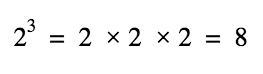# Log Node

## Description

Returns the logarithm of input In. Log is the inverse operation to the Exponential Node.

For example, the result of a base-2 Exponential using an input value of 3 is 8.Therefore the result of a base-2 Log using an input value of 8 is 3.

The logarithmic base can be switched between base-e, base-2 and base-10 from the Base dropdown on the node.

## Ports

Name Direction Type Description
In Input Dynamic Vector Input value
Out Output Dynamic Vector Output value

## Controls

Name Type Options Description
Base Dropdown BaseE, Base2, Base10 Selects the logarithmic base

## Generated Code Example

The following example code represents one possible outcome of this node per Base mode.

Base E

``````void Unity_Log_float4(float4 In, out float4 Out)
{
Out = log(In);
}
``````

Base 2

``````void Unity_Log2_float4(float4 In, out float4 Out)
{
Out = log2(In);
}
``````

Base 10

``````void Unity_Log10_float4(float4 In, out float4 Out)
{
Out = log10(In);
}
``````